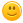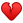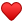# What's wrong with my code?

Hi there!I am studying at the university and I'm set to solve a program in C. Unfortunately, it doesn't work and neither my groupmates nor my teacher know why it doesn't work.I would be very grateful if you give me some advice on correcting my code. Thank you in advance!This is the task: s=(a2-p)/(b*log2(b+p)) if 2.5<=p<a and s=3.51*p1/2/(a-1).

#include <stdio.h>

#include <math.h>

#define a 3.651

#define b 5.82

main ( )

float p,s;

printf("Input p=");

scanf("f",&p);

if ((p>=2.5) && (p<a))

{

s=(a*a-p)/(b*(log(b+p)/log(a)));

printf("\ns=%f p=%f", s, p);

}

else

if ((p>4) && (p<=b))

{

s=(3.51*sqrt(p))/(a-1);

printf("\ns=%f p=%f", s, p);

}

}

+1 vote
answered Oct 13 by (5,120 points)
selected Oct 13 by Miafina

The issue is with scanf("f",&p); - Missing a % so should be scanf("%f",&p);

Not too sure on if the maths and logic is correct, however if the user enters a number that doesn't return true for the first if condition and the second if condition within the else, the code exits (might want to stop that).
commented Oct 13 by (400 points)
Hi Lios!
I don't know why  but in our reference book there is an example using scanf("f", &x) and they say this code is correct and works appropriately. Maybe it's a misprint.
Thanks again. Good luck in programming!
+1 vote
answered Oct 12 by (260 points)
hey! try this one...i think now it's working.

one of the warning is because you are using float in the program whereas main() is working in default way,which is int.

#include <stdio.h>
#include <math.h>
#define a 3.651
#define b 5.82
void main()
{
float p,s;
printf("Input p=");
scanf("%f",&p);
if ((p>=2.5) && (p<a))
{
s=(a*a-p)/(b*(log(b+p)/log(a)));
printf("\ns=%f p=%f", s, p);
}
else if((p>4) && (p<=b))
{
s=(3.51*sqrt(p))/(a-1);
printf("\ns=%f p=%f", s, p);
}
else     //just to define that p value is out of order
{
printf("please enter correct value of P");
}
}
commented Oct 13 by (400 points)
Hi Malik!
Your code is working appropriately and so is doing mine now). Thank you very much for your help.
Good luck in programming!